Thank you, Mike, for your ever-awesome explanations! Here’s a question from April 2017 SAT, Section 4.22:

The graphs in the xy-plane of the following quadratic equations each have x-intercepts of -2 and 4. The graph of which equation has its vertex farthest from the x-axis?

A) y = -7 (x + 2)(x – 4)

B) y = 1/10 (x + 2)(x – 4)

C) y = -1/2 (x + 2)(x – 4)

D) y = 5 (x + 2)(x – 4)

It’s useful to note that all these equations are the same after the leading coefficient. So, for example, we could just say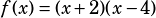and then the choices would just be:

A)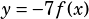B)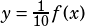C)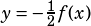D)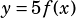The reason I point that out is that the effect of multiplying a function by a constant, in general, is that the larger the absolute value of the constant is, the more you’ll be stretching that function away from the x-axis. If you know that little fact, then you can immediately pick the answer choice with the coefficient with the largest absolute value, which is choice A.

If you don’t know that little fact, but you have a graphing calculator, you’re still in good shape. Just graph each one (on the same screen, if possible) to see which one has its vertex farthest from the x-axis.SAT Rockstar says: